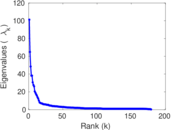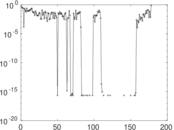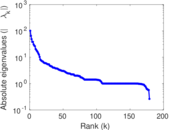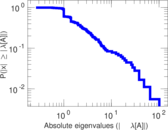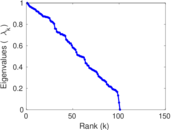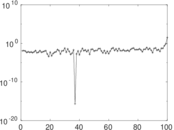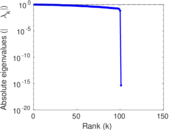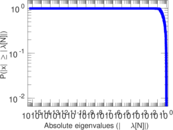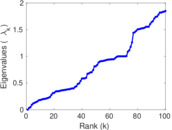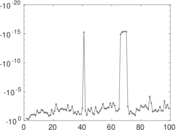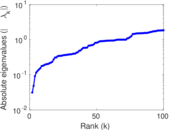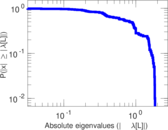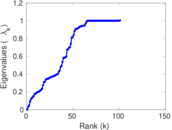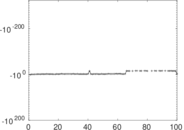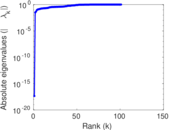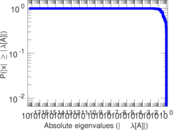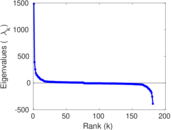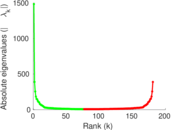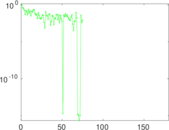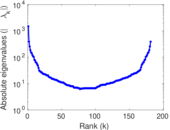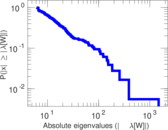# Wiktionary edits (su)

This is the bipartite edit network of the Sundanese Wiktionary. It contains users and pages from the Sundanese Wiktionary, connected by edit events. Each edge represents an edit. The dataset includes the timestamp of each edit.

 Code `msu` Internal name `edit-suwiktionary` Name Wiktionary edits (su) Data source http://dumps.wikimedia.org/ AvailabilityDataset is available for download Consistency checkDataset passed all tests Category Authorship network Dataset timestamp 2017-10-20 Node meaning User, article Edge meaning Edit Network formatBipartite, undirected Edge typeUnweighted, multiple edges Temporal dataEdges are annotated with timestamps

## Statistics

 Size n = 1,499 Left size n1 = 181 Right size n2 = 1,318 Volume m = 5,461 Unique edge count m̿ = 3,091 Wedge count s = 266,717 Claw count z = 20,750,762 Cross count x = 1,342,530,381 Square count q = 191,730 4-Tour count T4 = 2,608,842 Maximum degree dmax = 807 Maximum left degree d1max = 807 Maximum right degree d2max = 49 Average degree d = 7.286 19 Average left degree d1 = 30.171 3 Average right degree d2 = 4.143 40 Fill p = 0.012 957 0 Average edge multiplicity m̃ = 1.766 74 Size of LCC N = 1,252 Diameter δ = 12 50-Percentile effective diameter δ0.5 = 3.587 43 90-Percentile effective diameter δ0.9 = 5.716 14 Median distance δM = 4 Mean distance δm = 4.205 57 Gini coefficient G = 0.748 399 Balanced inequality ratio P = 0.207 013 Left balanced inequality ratio P1 = 0.109 504 Right balanced inequality ratio P2 = 0.285 479 Relative edge distribution entropy Her = 0.799 855 Power law exponent γ = 2.555 37 Tail power law exponent γt = 2.241 00 Tail power law exponent with p γ3 = 2.241 00 p-value p = 0.004 000 00 Left tail power law exponent with p γ3,1 = 1.641 00 Left p-value p1 = 0.238 000 Right tail power law exponent with p γ3,2 = 2.451 00 Right p-value p2 = 0.000 00 Degree assortativity ρ = −0.108 646 Degree assortativity p-value pρ = 1.396 51 × 10−9 Spectral norm α = 101.238 Algebraic connectivity a = 0.031 342 4 Spectral separation |λ1[A] / λ2[A]| = 1.559 40 Controllability C = 1,109 Relative controllability Cr = 0.755 965

## Plots

### Fruchterman–Reingold graph drawing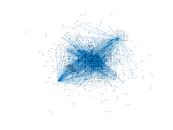### Degree distribution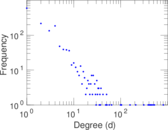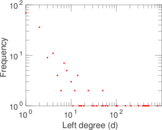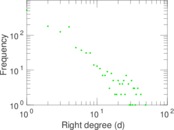### Cumulative degree distribution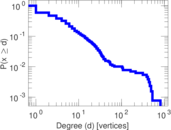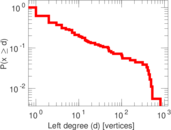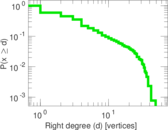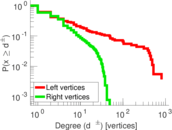### Lorenz curve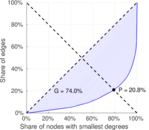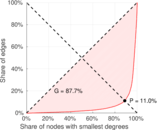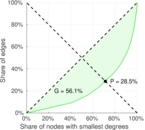### Spectral distribution of the adjacency matrix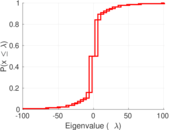### Spectral distribution of the normalized adjacency matrix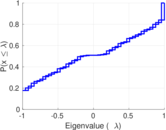### Spectral distribution of the Laplacian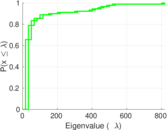### Spectral graph drawing based on the adjacency matrix### Spectral graph drawing based on the Laplacian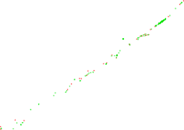### Spectral graph drawing based on the normalized adjacency matrix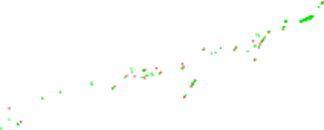### Degree assortativity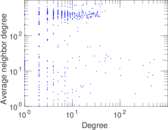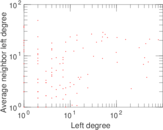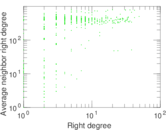### Zipf plot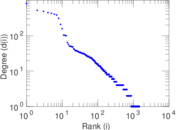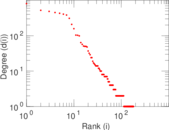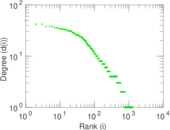### Hop distribution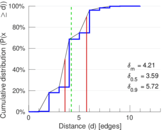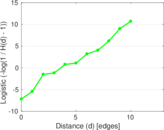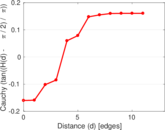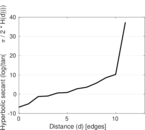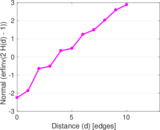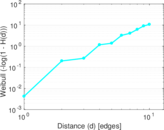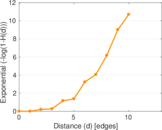### Double Laplacian graph drawing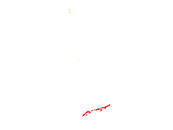### Delaunay graph drawing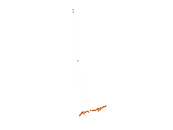### Edge weight/multiplicity distribution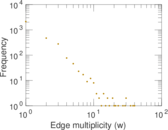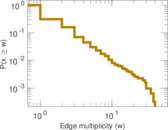### Temporal distribution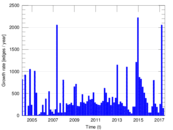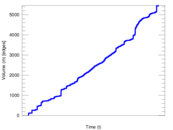### Temporal hop distribution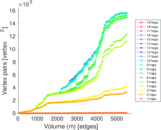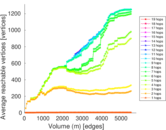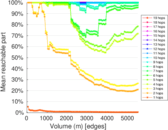### Diameter/density evolution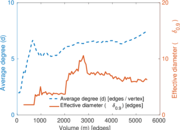### Matrix decompositions plots